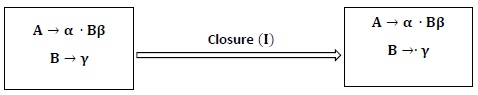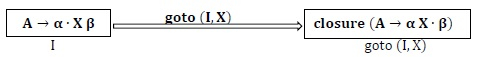# What is Canonical Collection of LR (0) items in compiler design?

The LR (0) item for Grammar G consists of a production in which symbol dot (.) is inserted at some position in R.H.S of production.

For Example − For the production S → ABC, the generated LR (0) items will be −

S →∙ ABC

S → A ∙ BC

S → AB ∙ C

S → ABC ∙

Production S → ε generates only one item, i.e., S →∙

Canonical LR (0) collection helps to construct LR parser called Simple LR (SLR) parser.

To create Canonical LR (0) collection for Grammar, 3 things are required −

• Augmented Grammar
• Closure Function
• goto Function

Augmented Grammar − If grammar G has start symbol S, then augmented Grammar is new Grammar G′ with new start symbol S′. Also, it will contain the production S′ → S.

Closure − For a Context-Free Grammar G, if I is the set of items or states of grammar G, then −

• Every item in I is in the closure (I).

• If rule A → α. B β is a rule in closure (I) and there is another rule for B such as B → γ then closure (I) will consist of A → α. Bβ and B → . γComputation of Closure

procedure closure (I)

• begin
• Repeat
• for each rule A→α∙B β in I and B→γ in G
• 𝐀𝐝𝐝 B→∙γ in I
• Until no more elements can be added to I;
• end
• goto (𝐈,𝐗): If there is a production A→α∙X β in I then goto (I,X) is defined as closure of the set of items of A→α X∙β where I is set of items and X is grammar symbol (non-terminal).Algorithm for construction of Canonical collection of sets of LR (0) items

• begin
• C = {closure (S′ → ∙ S)}
• Repeat
• For each set of elements I in C and each grammar symbol X such that goto (I, X) is not empty and is not in C
• add goto (I, X) to C
• Until no more sets of elements can be added to C
• end

Example − Consider the Grammar

B → Ba | b

Find closure (I) and goto (I)

Solution

Let B →∙ Ba

Since B appears after the dot. Therefore other production with B on L.H.S with the dot on starting of R.H.S of production will be added to closure, i.e., B →∙ B is also added to closure (I).

Closure(I) will be B → ∙ Ba                                         (1)

B → ∙ b                                                                           (2)

As, after the dot, we have B in (1)

goto(I, B) will be B → B ∙ a

∵ After Dot, we have b in (2)

goto(I, b) will be B → b ∙